Next: System with Specified Mean Up: Applications of Statistical Thermodynamics Previous: Canonical Probability Distribution

# Spin-1/2 Paramagnetism

The simplest microscopic system that we can analyze using the canonical probability distribution is one that has only two possible states. (There would clearly be little point in analyzing a system with only one possible state.) Most elements, and some compounds, are paramagnetic. In other words, their constituent atoms, or molecules, possess a permanent magnetic moment due to the presence of one or more unpaired electrons. Consider a substance whose constituent particles contain only one unpaired electron (with zero orbital angular momentum). Such particles have spin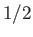[i.e., their spin angular momentum is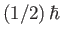], and consequently possess an intrinsic magnetic moment,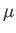. According to quantum mechanics, the magnetic moment of a spin-particle can point either parallel or antiparallel to an external magnetic field,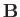. Let us determine the mean magnetic moment (parallel to),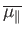, of the constituent particles of the substance when its absolute temperature is. We shall assume, for the sake of simplicity, that each atom (or molecule) only interacts weakly with its neighboring atoms. This enables us to focus attention on a single atom, and to treat the remaining atoms as a heat bath at temperature.

Our atom can be in one of two possible states. Namely, the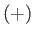state in which its spin points up (i.e., parallel to), or the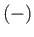state in which its spin points down (i.e., antiparallel to). In thestate, the atomic magnetic moment is parallel to the magnetic field, so that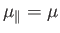. The magnetic energy of the atom is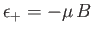. In thestate, the atomic magnetic moment is antiparallel to the magnetic field, so that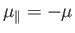. The magnetic energy of the atom is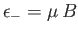.

According to the canonical distribution, the probability of finding the atom in thestate is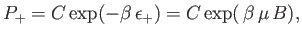(7.13)

where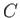is a constant, and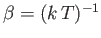. Likewise, the probability of finding the atom in thestate is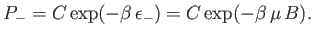(7.14)

Clearly, the most probable state is the state with the lower energy [i.e., thestate]. Thus, the mean magnetic moment points in the direction of the magnetic field (i.e., the atomic spin is more likely to point parallel to the field than antiparallel).

It is apparent that the critical parameter in a paramagnetic system is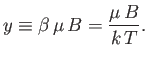(7.15)

This parameter measures the ratio of the typical magnetic energy of the atom,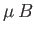, to its typical thermal energy,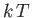. If the thermal energy greatly exceeds the magnetic energy then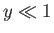, and the probability that the atomic moment points parallel to the magnetic field is about the same as the probability that it points antiparallel. In this situation, we expect the mean atomic moment to be small, so that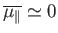. On the other hand, if the magnetic energy greatly exceeds the thermal energy then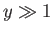, and the atomic moment is far more likely to be directed parallel to the magnetic field than antiparallel. In this situation, we expect.

Let us calculate the mean atomic moment,. The usual definition of a mean value gives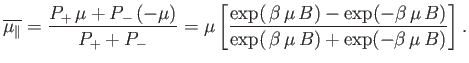(7.16)

This can also be written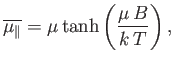(7.17)

where the hyperbolic tangent is defined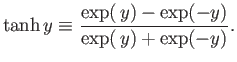(7.18)

For small arguments,,(7.19)

whereas for large arguments,,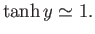(7.20)

It follows that at comparatively high temperatures,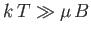,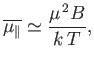(7.21)

whereas at comparatively low temperatures,,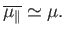(7.22)

Suppose that the substance contains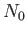atoms (or molecules) per unit volume. The magnetization is defined as the mean magnetic moment per unit volume, and is given by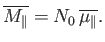(7.23)

At high temperatures,, the mean magnetic moment, and, hence, the magnetization, is proportional to the applied magnetic field, so we can write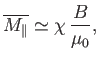(7.24)

where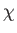is a dimensionless constant of proportionality known as the magnetic susceptibility, and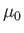the magnetic permeability of free space. It is clear that the magnetic susceptibility of a spin-1/2 paramagnetic substance takes the form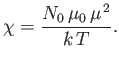(7.25)

The fact that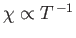is known as Curie's law, because it was discovered experimentally by Pierre Curie at the end of the nineteenth century. At low temperatures,,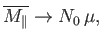(7.26)

so the magnetization becomes independent of the applied field. This corresponds to the maximum possible magnetization, in which all atomic moments are aligned parallel to the field. The breakdown of the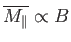law at low temperatures (or high magnetic fields) is known as saturation.Next: System with Specified Mean Up: Applications of Statistical Thermodynamics Previous: Canonical Probability Distribution
Richard Fitzpatrick 2016-01-25